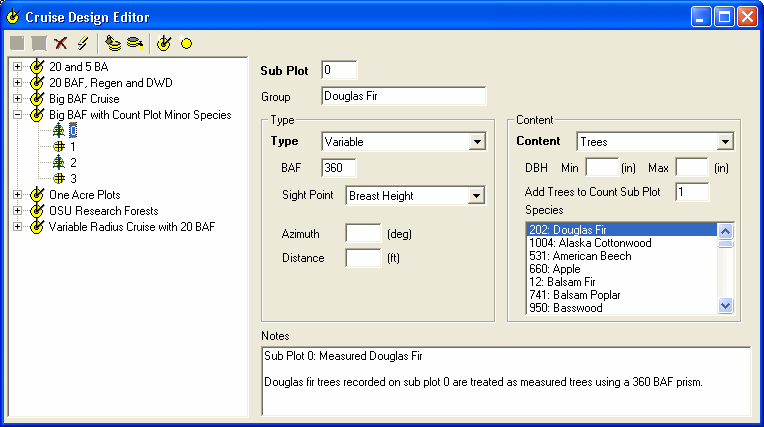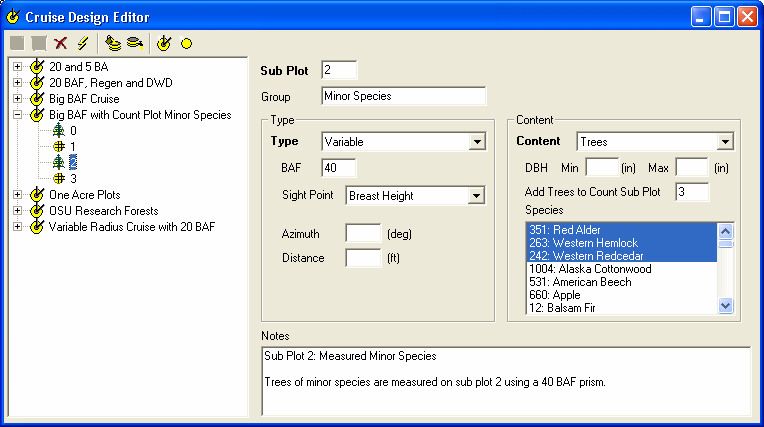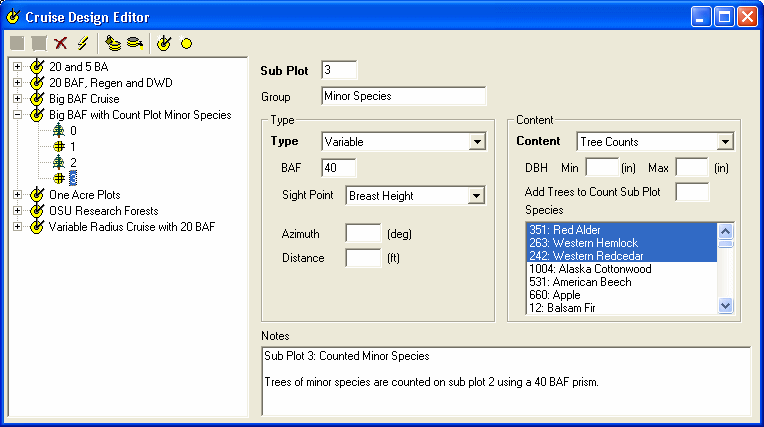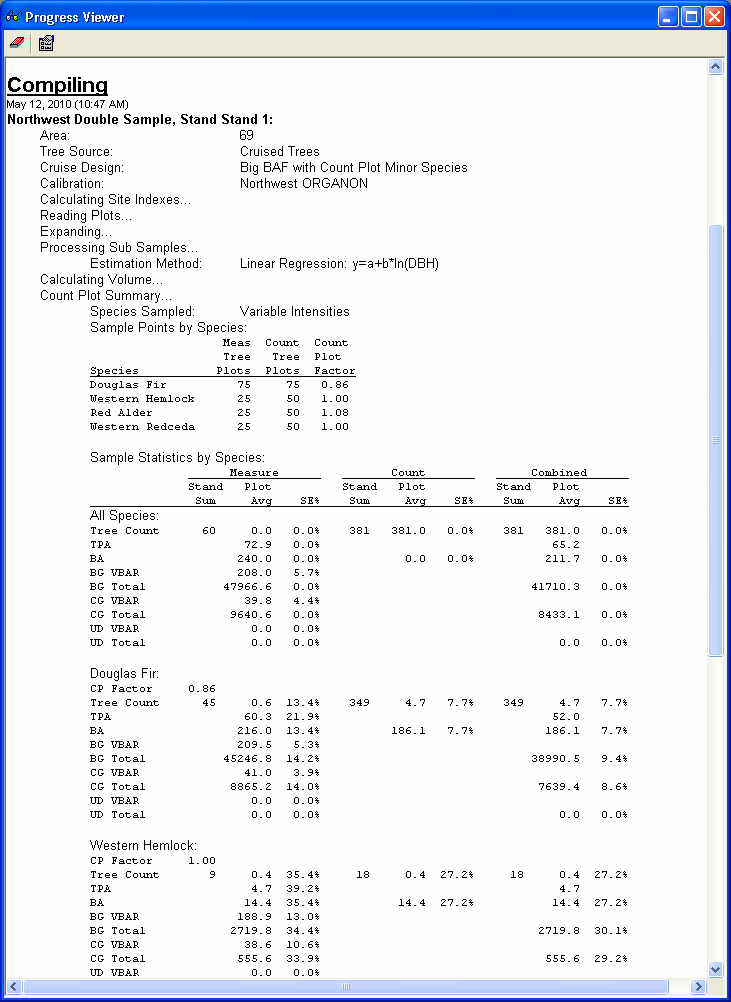# Double Sampling in Maintenance Release 2

Maintenance Release 2 of Assisi Inventory 2006 enhances support for double sampling in significant ways. You can now create sample designs that have Big BAF, Count Plot or both types of double sampling within a single cruise design. Species can be assigned to each type of double sample or to other non double sampled sub plots in your design. Measure trees are expanded using the count plot stats making count plot corrected tree lists available for other uses like growth and filtering.

### Double Sampling

Double sampling is a method where measurements with greater variability are sampled more intensely than measurements with less variability. This allows effort to be concentrated where it does more good. When the data is later compiled, the different sampling methods are combined in ways that produce better estimates than would be otherwise.

Assisi supports both 'Big BAF' and 'Count Plot' double sampling methods. The Big BAF method uses a big BAF prism to select trees for measure and a smaller BAF prism to select trees for counting. Both prisms are used at each sample point. The Count Plot method uses different prisms (or different fixed plot sizes) as well, but only one prism is used at each sample point.

### An Example

This sample design is a double sample where the major species (DF) is being sampled using a 'Big BAF' method and the minor species (RA, WH & RC) are being sampled using the 'Count Plot' method. The two methods are setup in Assisi using 4 sub plots: a measure and a count sub plot for DF and a measure and a count sub plot for the minor species. Because DF and minor species are being sampled using different methods, and thus different sampling intensities, the sample design must also state which species are being sampled on each sub plot.Sub Plot 0: Douglas fir trees recorded on sub plot 0 are treated as measured trees using a 360 BAF prism.Sub Plot 1: Douglas fir trees recorded on sub plot 1 are treated as count trees using a 40 BAF prism.Sub Plot 2: Trees of minor species are measured on sub plot 2 using a 40 BAF prism.Sub Plot 3: Trees of minor species are counted on sub plot 2 using a 40 BAF prism.

### The Field Data

Each tree record references one of the four sub plots depending on its species and whether it was measured or counted. Each plot (sample point) specifies which sub plots were actually in use at that point. For example, looking over the field data you will see that all plots are marked as having sub plots 0 and 1 in them. This is because DF was both measured and counted at each point. However, only about 1 of every 3 plots are marked with sub plot 2 and the other 2 of 3 plots are marked with sub plot 3. This is because minor species are measured on 1/3 of the points and counted on the other 2/3 of points.Plot 2004 states that sub plots 0, 1 and 2 were measured. This means measured and counts of DF were taken (sub plots 0 and 1) as well as measures of minor species (sub plot 2).Each tree states the sub plot it was collected on.

### The Calculations

Assisi calculates a volume to BA ratio (VBAR) using the measured trees and BA itself using the counted trees. The two are combined to get the estimated volume. The ratio of count to measure BA is also used to correct the per acre values for the measured trees so that they can be used for growth and filtering on reports.The Progress Viewer shows the count plot states as they are calculated. Stat are of course stored back to the database for reporting as well.